Common Ions and Complex Ions

Common Ions and Complex Ions

The Common-Ion Effect

When AgNO3 is added to a saturated solution of AgCl, it is often described as a source of a common ion, the Ag+ ion. By definition, a common ion is an ion that enters the solution from two different sources. Solutions to which both NaCl and AgCl have been added also contain a common ion; in this case, the Cl- ion. This section focuses on the effect of common ions on solubility product equilibria.

 Practice Problem 6:Calculate the solubility of AgCl in pure water. (AgCl: Ksp = 1.8 x 10-10) Click here to check your answer to Practice Problem 6 Click here to see a solution to Practice Problem 6

The common-ion effect can be understood by considering the following question: What happens to the solubility of AgCl when we dissolve this salt in a solution that is already 0.10 M NaCl? As a rule, we can assume that salts dissociate into their ions when they dissolve. A 0.10 M NaCl solution therefore contains 0.10 moles of the Cl- ion per liter of solution. Because the Cl- ion is one of the products of the solubility equilibrium, LeChatelier's principle leads us to expect that AgCl will be even less soluble in an 0.10 M Cl- solution than it is in pure water.

 Practice Problem 7:Calculate the solubility of AgCl in 0.10 M NaCl.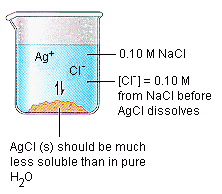Click here to check your answer to Practice Problem 7 Click here to see a solution to Practice Problem 7

Let's compare the results of the two above practice problems.

 In pure water: Cs = 1.3 x 10-5 M In 0.10 M NaCl: Cs = 1.8 x 10-9 M

These calculations show how the common-ion effect can be used to make an "insoluble" salt even less soluble in water.

The common ion effect can be applied to other equilibria, as well. Consider what happens when we add a generic acid (HA) to water. We now have two sources of a common ion the H3O+ ion.

HA(aq) + H2O(l)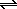H3O+(aq) + A-(aq)

2 H2O(l)H3O+(aq) + OH-(aq)

Thus, it isn't surprising that adding an acid to water decreases the concentration of the OH- ion in much the same way that adding another source of the Ag+ ion to a saturated solution of AgCl decreases the concentration of the Cl- ion.How to Keep a Salt From Precipitating

The common-ion effect can also be used to prevent a salt from precipitating from solution. Instead of adding a source of a common ion, we add a reagent that removes the common ion from solution.

Practice Problem 8:

Calculate the pH at which Cr(OH)3 just starts to precipitate from an 0.10 M Cr3+ solution. Let's use the results of this calculation to explain why it is impossible to prepare an 0.10 M Cr3+ solution at neutral pH.

 Cr(OH)3: Ksp = 6.3 x 10-31

The results of similar calculations for a number of different Cr3+ ion concentrations are given in the table below.

The Inverse Relationship Between the Equilibrium Concentrations of the Cr3+ and OH- Ions

 [Cr3+] (mol/L) [OH-] (mol/L) 1.0 8.6 x 10-11 1 x 10-1 1.8 x 10-10 1 x 10-2 4.0 x 10-10 1 x 10-3 8.6 x 10-10 1 x 10-4 1.8 x 10-9 1 x 10-5 4.0 x 10-9 1 x 10-6 8.6 x 10-9 1 x 10-7 1.8 x 10-8

Only a small fraction of these data will fit on a normal graph, such as the figure below.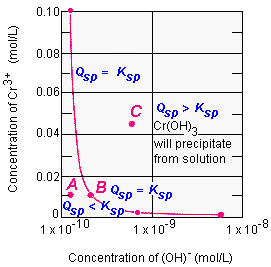We can overcome this problem by plotting the log of the Cr3+ ion concentrations versus the log of the OH- ion concentrations. The log [Cr3+] term for the data in the table below ranges from 0 to -7, and the log [OH-] term ranges from -7.7 to -10.1. Another way to overcome the problem involves plotting the Cr3+ and OH- concentrations on log-log graph paper. The figure below shows a log-log plot of the data in the above table.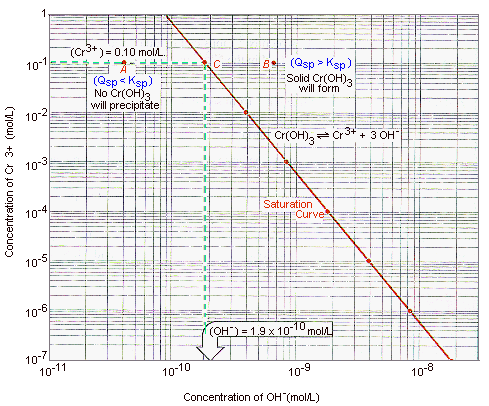The points along the solid line in the above figure represent combinations of Cr3+ and OH- ion concentrations at which the solution is saturated, and therefore at equilibrium. Point A represents a solution in which the OH- ion concentration is too small for Cr(OH)3 to precipitate when the Cr3+ ion concentration is 0.10 M. Point B corresponds to the set of conditions under which Cr(OH)3 just starts to precipitate. Point C describes a pair of Cr3+ and OH- ion concentrations for which the ion product is too large. Cr(OH)3 would precipitate from any solution that momentarily contained the Cr3+ and OH- ion concentrations that correspond to Point C.How To Separate Ions by Selective Precipitation

The concentration of an ion can be controlled to either prevent a solid from dissolving or to keep it in solution. The technique known as selective precipitation combines these processes to separate a mixture of two or more ions. It involves adding a reagent that selectively brings one of the ions out of solution as a precipitate while it leaves the other ions in the solution.

Some mixtures can be separated on the basis of the solubility rules. We can separate the Ag+ ion from a solution that contains the Cu2+ ion, for example, by adding a source of the Cl- ion. Silver chloride is an insoluble salt (Ksp = 1.8 x 10-10), which will precipitate from solution, whereas copper(II) chloride is soluble in water.

Selective precipitation becomes more of a challenge when the ions to be separated form salts with similar solubilities. Mn2+ and Ni2+ ions, for example, both form insoluble sulfides.

MnS: Ksp = 3 x 10-13

NiS: Ksp = 3.2 x 10-19

The 106-fold difference in the values of Ksp for these salts is large enough, however, to allow us to selectively precipitate the less soluble NiS salt from a mixture of these ions, without precipitating MnS as well.

 Practice Problem 9:Describe the conditions under which Ni2+ ions can be quantitatively precipitated as NiS from a solution that is 0.10 M in the Ni2+ and Mn2+ ions, while the Mn2+ ions are left in solution. Click here to check your answer to Practice Problem 9

The second criteria is easier to test than the first. It is relatively easy to determine when the S2- ion concentration is too largeand MnS starts to precipitate.

 Practice Problem 10:Calculate the S2- ion concentration at which MnS will begin to precipitate from a solution that is 0.10 M in Mn2+ ions.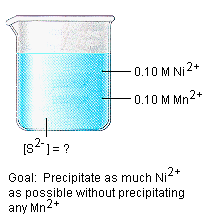Click here to check your answer to Practice Problem 10 Click here to see a solution to Practice Problem 10

We can therefore keep Mn2+ ions from precipitating from an 0.10 M solution if we can keep the S2- ion concentration smaller than 3 x 10-12 M. Let's see if this S2- ion concentration is large enough to effectively remove Ni2+ ions from the mixture.

 Practice Problem 11:Calculate the Ni2+ ion concentration in a solution to which enough S2- ion has been added to raise the concentration of this ion to 3 x 10-12 M. Click here to check your answer to Practice Problem 11 Click here to see an answer to Practice Problem 11

The 106-fold difference between the solubility products for MnS and NiS is large enough to allow us to separate the Mn2+ and Ni2+ ions in a mixture that is initially 0.10 M in both ions. Would this technique work equally well if the task involved separating the Mn2+ and Ni2+ ions when both concentrations were 0.010 M? Or when the Ni2+ ion was present at a 0.10 M concentration, but the Mn2+ concentration was only 0.0010 M? Instead of repeating the calculations in the above examples for each set of initial concentrations, we can construct a graph that allows us to answer this question for almost any combination of Mn2+ and Ni2+ ion concentrations.

The figure below shows the saturation curves for NiS and MnS plotted on the same piece of log-log graph paper.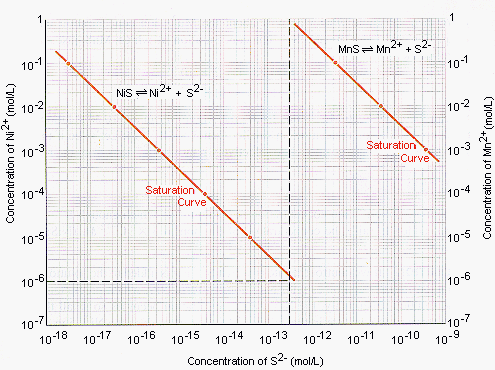The solid line at the left describes pairs of Ni2+ and S2- ion concentrations at which NiS is in equilibrium with these ions. The solid line at the right does the same for the equilibrium between MnS and the Mn2+ and S2- ions. The horizontal line at the bottom of the graph represents an Ni2+ ion concentration of 1 x 10-6 M. This line intersects the saturation curve for NiS at an S2- ion concentration of 3.2 x 10-13 M. The vertical line that represents this S2- ion concentration doesn't intersect the MnS saturation curve until the Mn2+ ion concentration is 1 M. This graph therefore tells us that as long as the initial concentrations of the Ni2+ and Mn2+ ions are less than 1 M, we can reduce the Ni2+ ion concentration to 1 x 10-6 M by adding S2- ions without precipitating MnS.How To Adjust the Concentration of an Ion

The preceding section leaves an important question unanswered: How do we adjust the S2- ion concentration in a solution so that it approaches but does not exceed 3 x 10-12 M? We start with an aqueous solution of the weak acidin this case, hydrogen sulfide dissolved in water.

 H2S(aq) + H2O(l)H3O+(aq) + HS-(aq) Ka1 = 1.0 x 10-7 HS-(aq) + H2O(l)H3O+(aq) + S2-(aq) Ka2 = 1.3 x 10-13

At room temperature, hydrogen sulfide is a gas that is only marginally soluble in water. (A saturated solution of this gas has a concentration of about 0.10 M at room temperature.) We can calculate the S2- ion concentration in a 0.10 M H2S solution.

[S2-] = Ka2 = 1.3 x 10-13 M

The S2- ion concentration in a saturated solution of H2S is therefore close to 3 x 10-12 M. How could we bring it even closer?

LeChatelier's principle suggests that we should be able to either increase or decrease the dissociation of this acid by adjusting the pH of the solution. If we add a strong acid, the amount of S2- ion should decrease as the acid-dissociation equilibria are driven toward the left. If we add a strong base, the S2- ion concentration should increase as the equilibria are pulled to the right.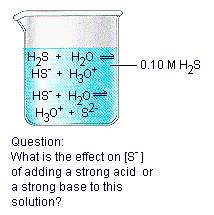In pure water, H2S dissociates by losing one proton at a time. But when we add either a strong acid or a strong base to this solution, the equilibria shift so much in one direction or the other that we can treat H2S as if it dissociates by losing two protons in a single step.

H2S(aq) + 2 H2O(l)2 H3O+(aq) + S2-(aq)

The equilibrium constant expression for the overall reaction is equal to the product of the equilibrium constant expressions for the individual steps.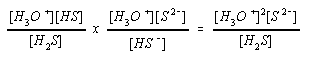The equilibrium constant for the overall reaction is therefore equal to the product of Ka1 times Ka2.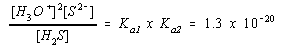A saturated solution of H2S has an initial concentration of 0.10 M. Because H2S is a weak acid, we can assume that the concentration of this acid at equilibrium is approximately equal to its initial concentration.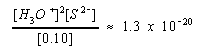We can then solve this approximate equation for the concentration of the H3O+ ion.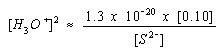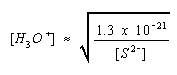Practice Problem 12:Calculate the pH to which a saturated solution of H2S in water must be adjusted for the S2- ion concentration to be 3 x 10-12 M. Click here to check your answer to Practice Problem 12 Click here to see a solution to Practice Problem 12

Combining the results of Practice Problem 12 with the calculations in the preceding section leads to the conclusion that we can separate the Ni2+ ion from the Mn2+ ion by adding a saturated solution of H2S that has been buffered at a pH of about 4.7. Most of the Ni2+ ion will precipitate as NiS, which can be collected by filtration. All of the Mn2+ ion will remain in solution.Complex Ions

The basic assumption behind the discussion of solubility equilibria is the idea that salts dissociate into their ions when they dissolve in water. Copper sulfate, for example, dissociates into the Cu2+ and SO42- ions in water.

 H2O CuSO4(s)Cu2+(aq) + SO42-(aq)

If we add 2 M NH3 to this solution, the first thing we notice is the formation of a light-blue, almost bluish-white, precipitate. This can be explained by combining what we know about acid-base and solubility equilibria. Ammonia acts as a base toward water to form a mixture of the ammonium and hydroxide ions

 NH3(aq) + H2O(l)NH4+(aq) + OH-(aq) Kb = 1.8 x 10-5

The OH- ions formed in this reaction combine with Cu2+ ions in the solution to form a Cu(OH)2 precipitate.

 Cu2+(aq) + 2 OH-(aq)Cu(OH)2(s) Ksp = 2.2 x 10-20

In theory, the OH- ion concentration should increase when more base is added to the solution. As a result, more Cu(OH)2 should precipitate from the solution. At first, this is exactly what happens. In the presence of excess ammonia, however, the Cu(OH)2 precipitate dissolves, and the solution turns deep-blue. This raises an important question: "Why does the Cu(OH)2 precipitate dissolve in excess ammonia?"

The first step toward answering this question involves writing the electron configuration of copper metal and its Cu2+ ion.

 Cu = [Ar] 4s1 3d10 Cu2+ = [Ar] 3d9

It is sometimes useful to think about the electron configuration of the Cu2+ ion in terms of the entire set of valence-shell orbitals. In addition to the nine electrons in the 3d subshell, this ion has an empty 4s orbital and a set of three empty 4p orbitals.

Cu2+ = [Ar] 4so 3d9 4po

The Cu2+ ion can therefore pick up pairs of nonbonding electrons from four NH3 molecules to form covalent Cu-N bonds,

Cu2+(aq) + 4 NH3(aq)Cu(NH3)42+(aq)

as shown in the figure below.Lewis Acid-Base Theory

G. N. Lewis was the first to recognize the similarity between this reaction and the acid-base reaction in which an H+ ion combines with an OH- ion to form a water molecule. Both reactions involve the transfer of a pair of nonbonding electrons from one atom to an empty orbital on another atom to form a covalent bond. Both reactions can therefore be interpreted in terms of an electron-pair acceptor combining with an electron-pair donor.

Lewis suggested that we could expand our definition of acids by assuming that an acid is any substance that acts like the H+ ion to accept a pair of nonbonding electrons. By definition, a Lewis acid is therefore an electron-pair acceptor. A Lewis base, on the other hand, is any substance that acts like the OH- ion to donate a pair of nonbonding electrons. A Lewis base is therefore an electron-pair donor.

The product of the reaction of a Lewis acid with a Lewis base is an acid-base complex. When the Cu2+ ion reacts with four NH3 molecules, the product of this reaction is called a complex ion.

 Cu2+(aq) + 4 NH3(aq)Cu(NH3)42+(aq) electron-pair acceptor (Lewis acid) electron-pair donor (Lewis base) acid-base complex or complex ion

Any atom, ion, or molecule with at least one empty valence-shell orbitals can be a Lewis acid. Any atom, ion, or molecule that contains at least one pair of nonbonding electrons is a Lewis base. All of the substances whose Lewis structures are shown in the figure below, for example, can act as Lewis bases to form complex ions.The Stepwise Formation of Complex Ions

When a transition-metal ion binds Lewis bases to form a coordination complex, or complex ion, it picks up these ligands one at a time. The Ag+ ion, for example, combines with NH3 in a two-step reaction. It first picks up one NH3 molecule to form a one-coordinate complex.

Ag+(aq) + NH3(aq)Ag(NH3)+(aq)

This intermediate then picks up a second NH3 molecule in a separate step.

Ag(NH3)+(aq) + NH3(aq)Ag(NH3)2+(aq)

It is possible to write equilibrium constant expressions for each step in these complex-ion formation reactions. The equilibrium constants for these reactions are known as complex-formation equilibrium constants, Kf. The equilibrium constant expressions for the two steps in the formation of the Ag(NH3)2+ complex ion are written as follows.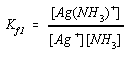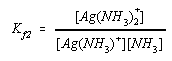The difference between the ease with which polyprotic acids lose the first and second protons is relatively large.

 H2S(aq) + H2O(l)H3O+(aq) + HS-(aq) Ka1 = 1.0 x 10-7 HS-(aq) + H2O(l)H3O+(aq) + S2-(aq) Ka2 = 1.3 x 10-13

As a result, calculations for polyprotic acids are based on the assumption of stepwise dissociation.

This assumption is harder to justify for complex formation equilibria. The difference between Kf1 and Kf2 for the complexes between Ag+ and ammonia, for example, is only a factor of 4.

 Ag+(aq) + NH3(aq)Ag(NH3)+(aq) Kf1 = 1.7 x 103 Ag(NH3)+(aq) + NH3(aq)Ag(NH3)2+(aq) Kf2 = 6.5 x 103

This means that most of the Ag+ ions that pick up one NH3 molecule to form the Ag(NH3)+ complex ion are likely to pick up another NH3 to form the two-coordinate Ag(NH3)2+ complex ion. The table below summarizes the concentrations of the Ag+, Ag(NH3)+, and Ag(NH3)2+ ions over a range of NH3 concentrations.

The Effect of Changes in the NH3 Concentration on the
Fraction of Silver Present as the Ag+, Ag(NH3)+, or Ag(NH3)2+ Ions

 [NH3] (M) Ag+ (%) Ag(NH3)+ (%) Ag(NH3)2+ (%) 10-6 99.8 0.2 0.001 10-5 98.2 1.7 0.1 10-4 78.1 13.3 8.6 10-3 7.3 12.4 80.4 10-2 0.09 1.5 98.4 10-1 0.0009 0.2 99.8

These data plotted in the figure below.Essentially all of the silver is present as the Ag+ ion at very low concentrations of NH3. As the NH3 concentration increases, the dominant species soon becomes the two-coordinate Ag(NH3)2+ ion. Even at NH3 concentrations as small as 0.0010 M, most of the silver is present as the Ag(NH3)2+ ion.

The concentration of the one-coordinate Ag(NH3)+ intermediate is never very large. Either the NH3 concentration is so small that most of the silver is present as the Ag+ ion or it is large enough that essentially all of the silver is present as the two-coordinate Ag(NH3)2+ complex ion.

If the only important components of this equilibrium are the free Ag+ ion (at low NH3 concentrations) and the two-coordinate Ag(NH3)2+ complex ion (at moderate to high NH3 concentrations), we can collapse the individual steps in this reaction into the following overall equation.

Ag+(aq) + 2 NH3(aq)Ag(NH3)2+(aq)

The overall complex formation equilibrium constant expression for this reaction is written as follows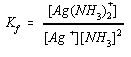This expression is equal to the product of the equilibrium constant expressions for the individual steps in the reaction.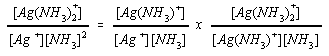The overall complex formation equilibrium constant is therefore equal to the product of the Kf values for the individual steps.

Kf = Kf1 x Kf2 = 1.1 x 107

Practice Problem 13:

Calculate the complex formation equilibrium constant for the two-coordinate Fe(SCN)2+ complex ion from the following data.

 Fe3+(aq) + SCN-(aq)Fe(SCN)2+(aq) Kf1 = 890 Fe(SCN)2+(aq) + SCN-(aq)Fe(SCN)2+(aq) Kf2 = 2.6Complex Dissociation Equilibrium Constants

Complex ions can also be described in terms of complex dissociation equilibria. We can start by assuming, for example, that most of the silver ions in an aqueous solution are present as the two-coordinate Ag(NH3)2+ complex ion. We then assume that some of these ions dissociate to form Ag(NH3)+ complex ions and then eventually Ag+ ions.

Ag(NH3)2+(aq)Ag(NH3)+(aq) + NH3(aq)

Ag(NH3)+(aq)Ag+(aq) + NH3(aq)

A complex dissociation equilibrium constant (Kd) expression can be written for each of these reactions.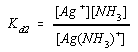Alternatively, the individual steps in this reaction can be collapsed into an overall equation, which can be described by an overall equilibrium constant expression.

Ag(NH3)2+(aq)Ag+(aq) + 2 NH3(aq)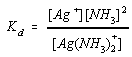Practice Problem 14:Calculate the complex dissociation equilibrium constant for the Cu(NH3)42+ ion from the value of Kf for this complex. [Cu(NH3)42+: Kf = 2.1 x 1013] Click here to check your answer to Practice Problem 14 Click here to see a solution to Practice Problem 14Approximate Complex Ion Calculations

Example: Let's determine what fraction of the total iron(III) concentration is present as the Fe3+ ion in a solution that was initially 0.10 M Fe3+ and 1.0 M SCN-?

 Fe(SCN)2+: Kf = 2.3 x 103

Complex-formation equilibria provide another example of the general rule that it is useful to begin equilibrium calculations by comparing the reaction quotient for the initial conditions with the equilibrium constant for the reaction. The initial conditions can be summarized as follows.

 Fe3+(aq) + 2 SCN-(aq)Fe(SCN)2+(aq) Kf = 2.3 x 103 Initial: 0.10 M 1.0 M 0

The initial value of the reaction quotient is therefore equal to zero.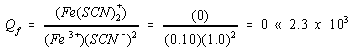Because the reaction quotient is very much smaller than the equilibrium constant for the reaction, it would be absurd to assume that the reaction is close to equilibrium. A way around this problem is to define a set of intermediate conditions in which we drive the reaction as far as possible toward the right.

 Fe3+(aq) + 2 SCN-(aq)Fe(SCN)2+(aq) Kf = 2.3 x 103 Initial: 0.10 M 1.0 M 0 Change: - 0.10 M - 2(0.010) M + 0.10 M Intermediate: 0 0.8 M 0.10 M

We then assume that the reaction comes to equilibrium from these intermediate conditions.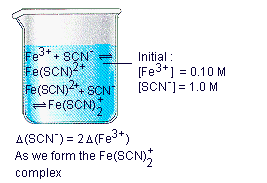Fe3+(aq) + 2 SCN-(aq)Fe(SCN)2+(aq) Kf = 2.3 x 103 Intermediate: 0 0.8 M 0.10 M Equilibrium:C 0.8 + 2C 0.10 -C

Substituting this information into the equilibrium expression for the reaction gives the following equation.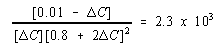We are now ready to assume thatC is relatively small.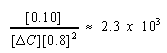Solving this approximate equation gives the following result.C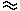6.8 x 10-5

The assumption thatC is relatively small is valid because the problem was defined so that it would be valid. We can now use this value of ? to answer the original question.

[Fe3+] = 6.8 x 10-5 M

Even though the complex formation equilibrium constant is not very large, essentially all of the iron in this solution is present as the Fe(SCN)2+ complex ion. Only a negligible fraction is present as the Fe3+ ion.Practice Problem 15:Calculate the concentration of the Cu2+ ion in a solution that is initially 0.10 M Cu2+ and 1.0 M NH3.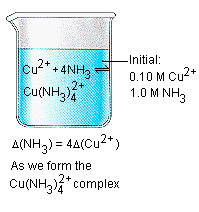Click here to check your answer to Practice Problem 15 Click here to see a solution to Practice Problem 15

The results of the preceding example can be used to explain why Cu(OH)2 dissolves in excess ammonia. Before we can do this, however, we need to understand why Cu(OH)2 precipitates in the first place. Ammonia acts as a base toward water.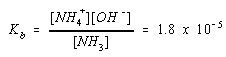Even fairly dilute solutions of the OH- ion have more than enough OH- ion to precipitate Cu(OH)2 from an 0.10 M Cu2+ ion solution. As the amount of NH3 added to the solution increases, the concentration of the OH- ion increases. But it doesn't become very much larger. The OH- ion concentration in an 0.001 M NH3 solution is 1.3 x 10-4 M. By the time the NH3 concentration reaches 0.10 M, the OH- ion concentration has increased by only a factor of 30, to 4.2 x 10-3 M.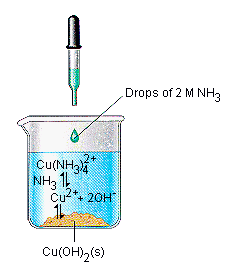As the amount of NH3 added to the solution increases, the concentration of Cu2+ ions rapidly decreases because these ions are tied up as Cu(NH3)42+ complex ions. According to the preceding exercise, the Cu2+ ion concentration in 1.0 M NH3 is only 3.7 x 10-14 M. Thus, the ion product for Cu(OH)2 under these conditions is about the same size as the solubility product for this compound.

Qsp = (Cu2+)(OH-)2 = (3.7 x 10-14)(4.2 x 10-3)2 = 6.5 x 10-19Ksp

As soon as the NH3 concentration exceeds 1 M, the Cu2+ ion concentration becomes so small that the ion product for Cu(OH)2 is smaller than Ksp, and the Cu(OH)2 precipitate dissolves.Using Complex Ion Equilibria to Dissolve an Insoluble Salt

The key to using complex-ion equilibria to dissolve an insoluble salt is simple: Choose a complex for which Kf is large enough that the concentration of the uncomplexed metal ion is too small for the ion product to exceed the solubility product.

Practice Problem 16:

Calculate the solubility of AgBr in 1 M S2O32-.

 AgBr: Ksp = 5 x 10-13 Ag(S2O3)23-: Kf = 2.9 x 1013